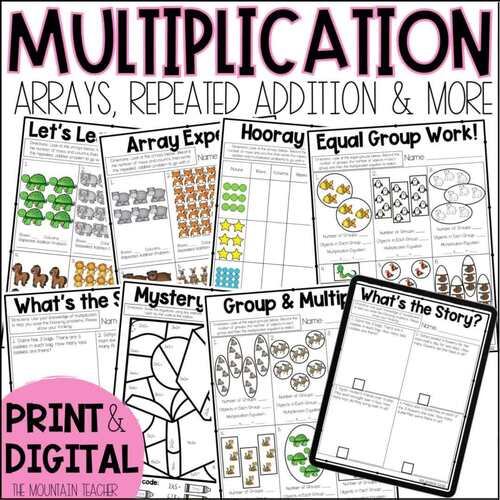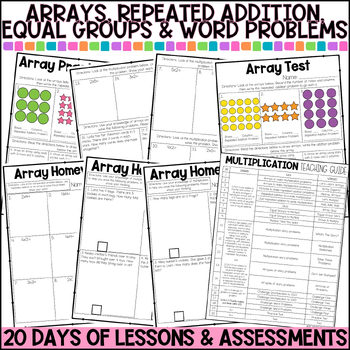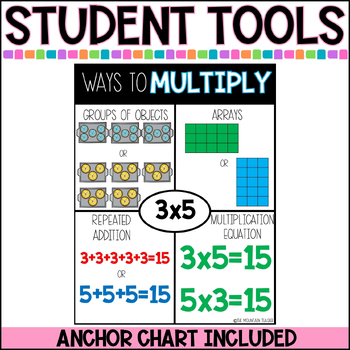# Multiplication Practice Worksheets with Arrays, Repeated Addition & Equal Groups

Rated 4.76 out of 5, based on 37 reviews
37 Ratings;
1st - 3rd
Subjects
Resource Type
Standards
Formats Included
• PDF
•Google Apps™
Pages
45+
Report this resource to TPTThe Teacher-Author indicated this resource includes assets from Google Workspace (e.g. docs, slides, etc.).
Easel Activity Included
This resource includes a ready-to-use interactive activity students can complete on any device.  Easel by TPT is free to use! Learn more.

#### What educators are saying

I used this and prepped this for my students to use at the end of the year for review and to send to summer school.
This was a very helpful resource to use with students who are just being introduced to multiplication. Thank you!
##### Also included in
1. Planning your 2nd grade math lessons for the year is EASY with this YEAR LONG bundle that includes 40 weeks of 2nd grade math worksheets, activities (printable and digital), homework, pacing guides and assessments for every common core state standard. What's Included With the 2nd Grade Math Workshee
Price \$69.99Original Price \$73.92Save \$3.93

### Description

Teach multiplication using repeated addition, arrays, equal groupings and word problems to your 2nd or 3rd graders with ease using these multiplication practice worksheets and assessments. This 4 week unit is filled with printable and digital multiplication practice worksheets, assessments and activities covering repeated addition, arrays, equal groupings, word problems and 4 challenge activities.

What's Included With the Multiplication Practice Worksheets Pack:

• 17 Multiplication Practice Worksheets
• 2 Multiplication Tests (practice and post test)
• 1 Multiplication Quiz
• 3 Multiplication Homework Worksheets
• Teaching Guide
• Anchor Chart (multiplication with repeated addition, equal groups and arrays)

Multiplication Practice Worksheets Pack Formats:

• Multiplication Practice Worksheets - Printable in Black and White or Color
• TpT Easel Activity

The Multiplication Practice Worksheets Cover the Following Standards:

• 2.OA.C.4: Use addition to find the total number of objects arranged in rectangular arrays with up to 5 rows and up to 5 columns; write an equation to express the total as a sum of equal addends.
• 2.OA.A.1: Use addition and subtraction within 100 to solve one- and two-step word problems involving situations of adding to, taking from, putting together, taking apart, and comparing, with unknowns in all positions, e.g., by using drawings and equations with a symbol for the unknown number to represent the problem.

Check Out My Other Second Grade Math Units:

- Time

OR check out my Second Grade Let's Do Math Curriculum, including DAILY warm ups, turn and talks, mini lessons, weekly quizzes, unit assessments, benchmark tests, daily worksheets and so much more.

You can also connect with me for first access to my resources, giveaways and FREEBIES via: Email | Website | Instagram | Facebook | Teachers Pay Teachers

Enjoy!

Total Pages
45+
Included
Teaching Duration
1 month
Report this resource to TPT
Reported resources will be reviewed by our team. Report this resource to let us know if this resource violates TPT’s content guidelines.

### Standards

to see state-specific standards (only available in the US).
Use addition to find the total number of objects arranged in rectangular arrays with up to 5 rows and up to 5 columns; write an equation to express the total as a sum of equal addends.
Interpret products of whole numbers, e.g., interpret 5 × 7 as the total number of objects in 5 groups of 7 objects each. For example, describe a context in which a total number of objects can be expressed as 5 × 7.
Solve two-step word problems using the four operations. Represent these problems using equations with a letter standing for the unknown quantity. Assess the reasonableness of answers using mental computation and estimation strategies including rounding.